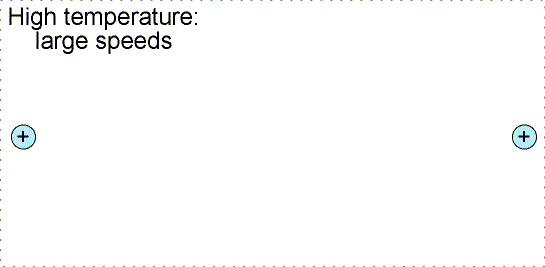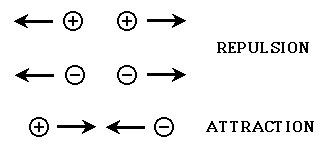×#### Thank you for registering.

One of our academic counsellors will contact you within 1 working day.

Click to Chat

1800-1023-196

+91-120-4616500

CART 0

• 0

MY CART (5)

Use Coupon: CART20 and get 20% off on all online Study Material

ITEM
DETAILS
MRP
DISCOUNT
FINAL PRICE
Total Price: Rs.

There are no items in this cart.
Continue Shopping• Complete Physics Course - Class 11
• OFFERED PRICE: Rs. 2,968
• View Details

Nuclear Forces

Table of Content

In a typical Nuclear Reaction

Related ResourcesThe protons and neutrons are held together by the strong attractive forces inside the nucleus. These forces are called as nuclear forces.These forces, which act between the nucleons, are mainly responsible for the stability of the nucleus. Various characteristics of nuclear forces are given below:

(a) Nuclear forces are attractive in nature:- The magnitude which depends upon inter nucleon distance is of very high order.

(b) Nuclear forces are charge independent:- Nature of force remains the same whether we consider force between two protons, between two neutrons or between a proton and a neutron.

(c) These are short range forces:- Nuclear forces operate between two nucleons situated in close neighborhood only.

(d) Nuclear forces decrease very quickly with distance between two nucleons:- Their rate of decrease is much rapid than that of inverse square law forces. The forces become negligible when the nucleons are more than 10-12 cm apart.

(v) Nuclear forces are spin dependent:- Nucleons having parallel spin are more strongly bound to each other than those having anti-parallel spin.The Strong Nuclear Force (also referred to as the strong force) is one of the four basic forces in nature (the others being gravity, the electromagnetic force, and the weak nuclear force). As its name implies, it is the strongest of the four. However, it also has the shortest range, meaning that particles must be extremely close before its effects are felt. Its main job is to hold together the subatomic particles of the nucleus (protons, which carry a positive charge, and neutrons, which carry no charge. These particles are collectively called nucleons). As most people learn in their science education, like charges repel (+ +, or - -), and unlike charges attract (+ -).If you consider that the nucleus of all atoms except hydrogen contain more than one proton, and each proton carries a positive charge, then why would the nuclei of these atoms stay together? The protons must feel a repulsive force from the other neighboring protons. This is where the strong nuclear force comes in. The strong nuclear force is created between nucleons by the exchange of particles called mesons. As long as this meson exchange can happen, the strong force is able to hold the participating nucleons together.The nucleons must be extremely close together in order for this exchange to happen. The distance required is about the diameter of a proton or a neutron. If a proton or neutron can get closer than this distance to another nucleon, the exchange of mesons can occur, and the particles will stick to each other. If they can't get that close, the strong force is too weak to make them stick together, and other competing forces (usually the electromagnetic force) can influence the particles to move apart. This is represented in the following graphic. The dotted line surrounding the nucleon being approached represents any electrostatic repulsion that might be present due to the charges of the nucleons/particles that are involved. A particle must be able to cross this barrier in order for the strong force to "glue" the particles together.In the case of approaching protons/nuclei, the closer they get, the more they feel the repulsion from the other proton/nucleus (the electromagnetic force). As a result, in order to get two protons/nuclei close enough to begin exchanging mesons, they must be moving extremely fast (which means the temperature must be really high), and/or they must be under immense pressure so that they are forced to get close enough to allow the exchange of meson to create the strong force. Now, back to the nucleus. One thing that helps reduce the repulsion between protons within a nucleus is the presence of any neutrons. Since they have no charge they don't add to the repulsion already present, and they help separate the protons from each other so they don't feel as strong a repulsive force from any other nearby protons. Also, the neutrons are a source of more strong force for the nucleus since they participate in the meson exchange. These factors, coupled with the tight packing of protons in the nucleus so that they can exchange mesons creates enough strong force to overcome their mutual repulsion and force the nucleons to stay bound together. The preceding explanation shows the reason why it is easier to bombard a nucleus with neutrons than with protons. Since the neutrons have no charge, as they approach a positively charged nucleus they will not feel any repulsion. They therefore can easily "break" the electrostatic repulsion barrier to being exchanging mesons with the nucleus, thus becoming incorporated into it.

Refer this video to know more about nuclear forces:-

In a typical Nuclear Reaction,

(i) In nuclear reactions, sum of masses before reaction is greater than the sum of masses after the reaction. The difference in masses appears in form of energy following the Law of inter-conversion of mass & energy. The energy released in a nuclear reaction is called as Q Value of a reaction and is given as follows.

If difference in mass before and after the reaction is Δm amu (Δm = mass of reactants minus mass of products) then Q value = Δm (931) MeV

(ii) Law of conservation of momentum is also followed.

(iii) Total number of protons and neutrons should also remain same on both sides of a nuclear reaction.Nuclear forces exist in small region (of diameter 10–15 m = 1 fm). The nuclear force between two nucleons decrease rapidly as the separation between them increases and becomes negligible at separation more than 10 fm.

Nuclear force are much stronger than electromagnetic force or gravitational attractive forces.

The nuclear force between two proton is same as that between two neutrons or between a neutron and proton. This is known as charge independent character of nuclear forces.

The force, while stronger than the electrical force, needed to operate only within a short range. Otherwise it would attract protons at any distance.

The force needed to become neutral at even shorter distances. Otherwise protons would be forced together, possibly extinguishing themselves as an electron and positron are said to do.

The force also needed to work on neutrons which have neutral electric charge. Thus the possibility exists that the force should probably affect all subatomic particles and also cause electrons to cling together.

An -particle accelerated through V volt is fired towards a nucleus. Its distance of closest approach is r. If a proton accelerated through the same potential is fired towards the same nucleus, the distance of closest approach of proton will be xr. Find value of x.

Solution:-

In accordance to law of conservation energy, decrease in kinetic energy will be seen in the form of increase in potential energy.

So, qV = (1/4πε0) (Qq/r)

Or, r = Q/[(4πε0)V]

From the above observation, we conclude that, r is independent of q.

___________________________________________________________________________________________

A radioactive source in the form of metal sphere of diameter 3.210-3 m emits -particles at constant rate of 6.251010particles/sec. The source is electrically insulated and all the -particles are emitted from the surface. Calculate the time, for which the potential of the sphere will rise to 1V.

Solution:-

As, (1/4πε0) (q/R) = V

So, net/4πε0R = V

Or, t = 4πε0RV/ne

t = (3.210-31)/(2910962510101.610-19)

=17.710-6s

= 18µs

_______________________________________________________________________________________

Problem 3:-

Calculate the Q-value of the nuclear reaction:

0C12  10Ne20 + 2He4

The following data are given:

m(6C12) = 12.000000 u

m(10Ne20) = 12.000000 u

m(2Ne4) = 4.002603 u

Solution:-

The Q-value of this reaction may be easily calculated may be easily calculated from the masses of the individual nuclei.

Q = [2m (6C12) – {m (10Ne20) + m (2He4)}]e2

=24.000000 – (19.992439 + 4.002603)} u × c2

=4.618 MeV

__________________________________________________________________________________________

Problem 4:-

Binding energy of the calculate the mass defect and the binding energy of an α-particle given the following date:

mn = 1.0086665 u

mp = 1.007825 u

mα = 4.0026 u

(1u = 931.5 MeV/c2)

Solution:-

The mass defect of an α-particle is given by

Δm = (2 × 1.008665 + 2 × 1.007825) – 4.0026)u

= 0.03038u

The Binding energy is related to the mass defect by the reaction.

B.E. = Δm.c2

= 0.03038 × 931.5 MeV

= 28.3 MeV (approximately)Question 1:-

Of the three basic gravitational electrostatic and nuclear, which two are able to provide an attractive force between two neutrons:

(a) Electrostatic and gravitational           (b) Electrostatic and nuclear

(c) Gravitational and nuclear                  (d) Some of the forces like vander Walls.

Question 2:-

The constituents of the nucleus are held together by

(a) electromagnetic force             (b) weak force

(c) strong force                              (d) gravitational force

Question 3:-

The protons are able to exist in the nucleus of an atom, because

(a) strong attractive nuclear forces bind them

(b) attractive electrical forces act between them

(c) the repulsive forces between the positive charges on the protons is small at small distances.

(d) of nuclear fusion forces.

Question 4:-

The ionization energy of an atom compared to the binding energy of a nucleus is:

(a) more               (b) less

(c) equal               (d) sometimes more and sometimes less.

Question 5:-

When do two protons attract each other? When:

(a) the distance between them is 10-10 cm              (b) the distance between them is 10-1 cm

(c) the distance between them is 10-15 cm               (d) this will never happen.Q.1
Q.2
Q.3
Q.4
Q.5

c

b

a

b

c

Related Resources:-

You might like to refer Mass Defect and Binding Energy.

For getting an idea of the type of questions asked, refer the  Previous Year Question Papers.### Course Features

• 101 Video Lectures
• Revision Notes
• Previous Year Papers
• Mind Map
• Study Planner
• NCERT Solutions
• Discussion Forum
• Test paper with Video Solution